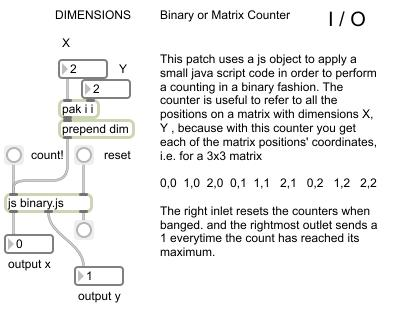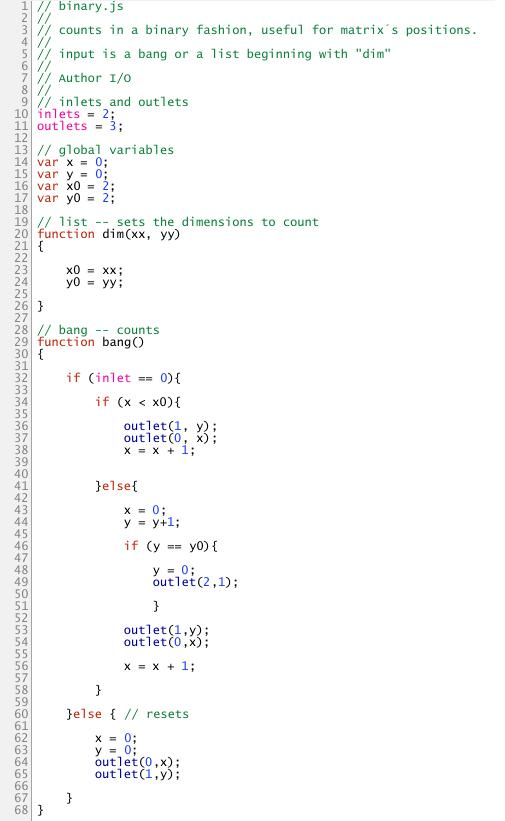# Counter in Max MSP using Java ScriptSo… I want to use Jitter to create objects and distribute them in the visual virtual space, and I need to create matrices with as many locations as objects I want to display.

These objects are to be assigned coordinates and dimensions. Since the objects will be distributed on a circular-like manner, I want to go through each position of the matrix and give it X and Y coordinates.

To address each of the positions of the matrix, (0,0   0,1, 0,2 etc) I decided to create a small routine using Java Script, since I was having problems with the logic in the patcher.

So, the small patch here contains a js object with a Java Script routine in it. This increases its count with every bang it receives, outputing the current values through the first two outlets, and outputing a 1 through the rightmost outlet when the count has reached its maximum.

The format of the counting is binary like, increasing x first until reaching its maximum, then reseting x and increasing y by one, after which x is incremented again until reaching its maximum and so to increas y again by one. This continues until both maximums have been reached.

The dimensions of the count can be modified by sending the message “dim x y” where X and Y are integers.

Here is the script: